# RD Sharma Solutions for Class 9 Maths Chapter 20 Surface Area and Volume of A Right Circular Cone Exercise 20.1

Class 9 Chapter 20 – Surface Area and Volume of A Right Circular Cone Exercise 20.1 solutions are provided here. These solutions have been prepared by our experts and they have provided accurate answers to all the exercise questions which students can refer and be ready to solve questions at the time of their examinations. This chapter of RD Sharma Class 9  introduces students to a lot of questions related to the surface area of a right circular cone.

### Download PDF of RD Sharma Solutions for Class 9 Maths Chapter 20 Surface Area and Volume of A Right Circular Cone Exercise 20.1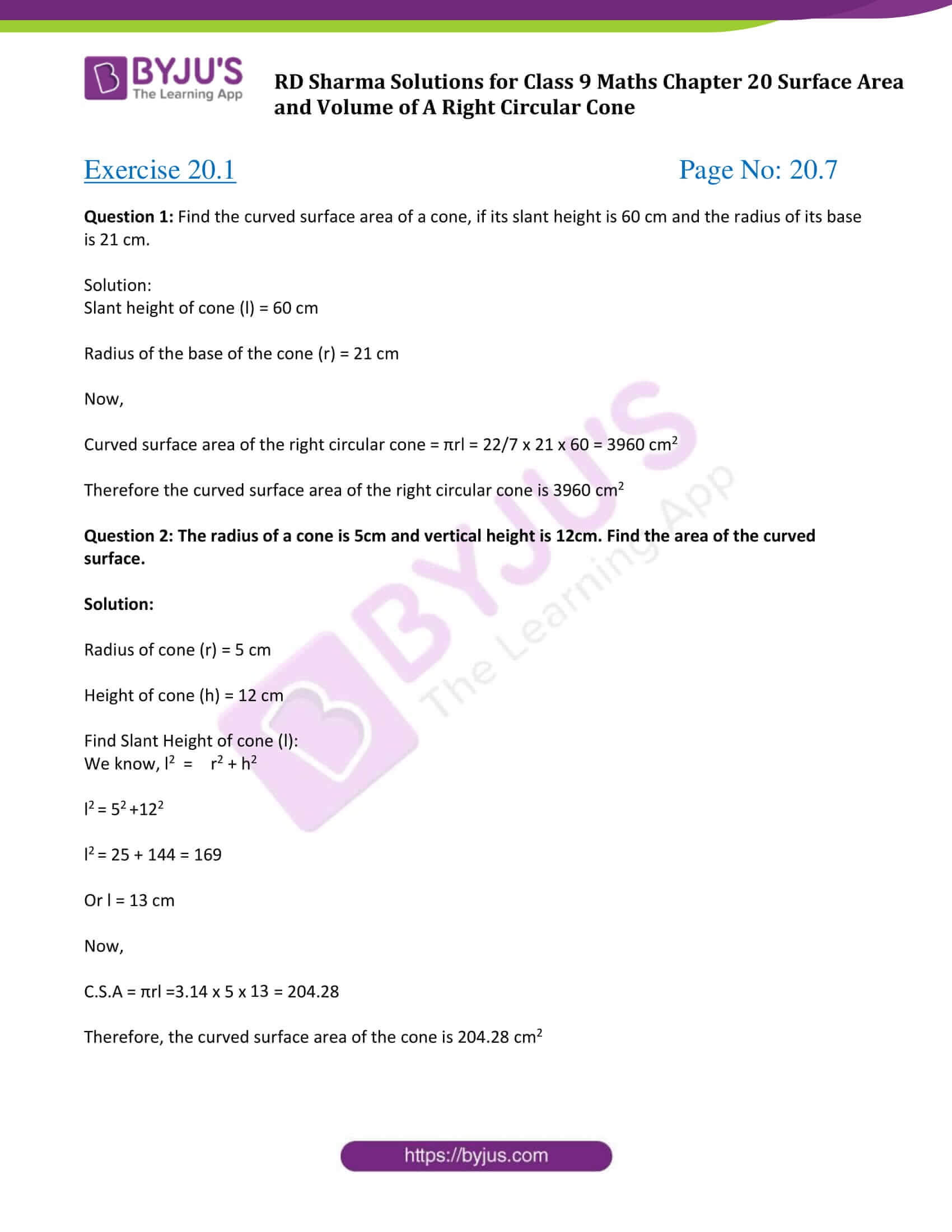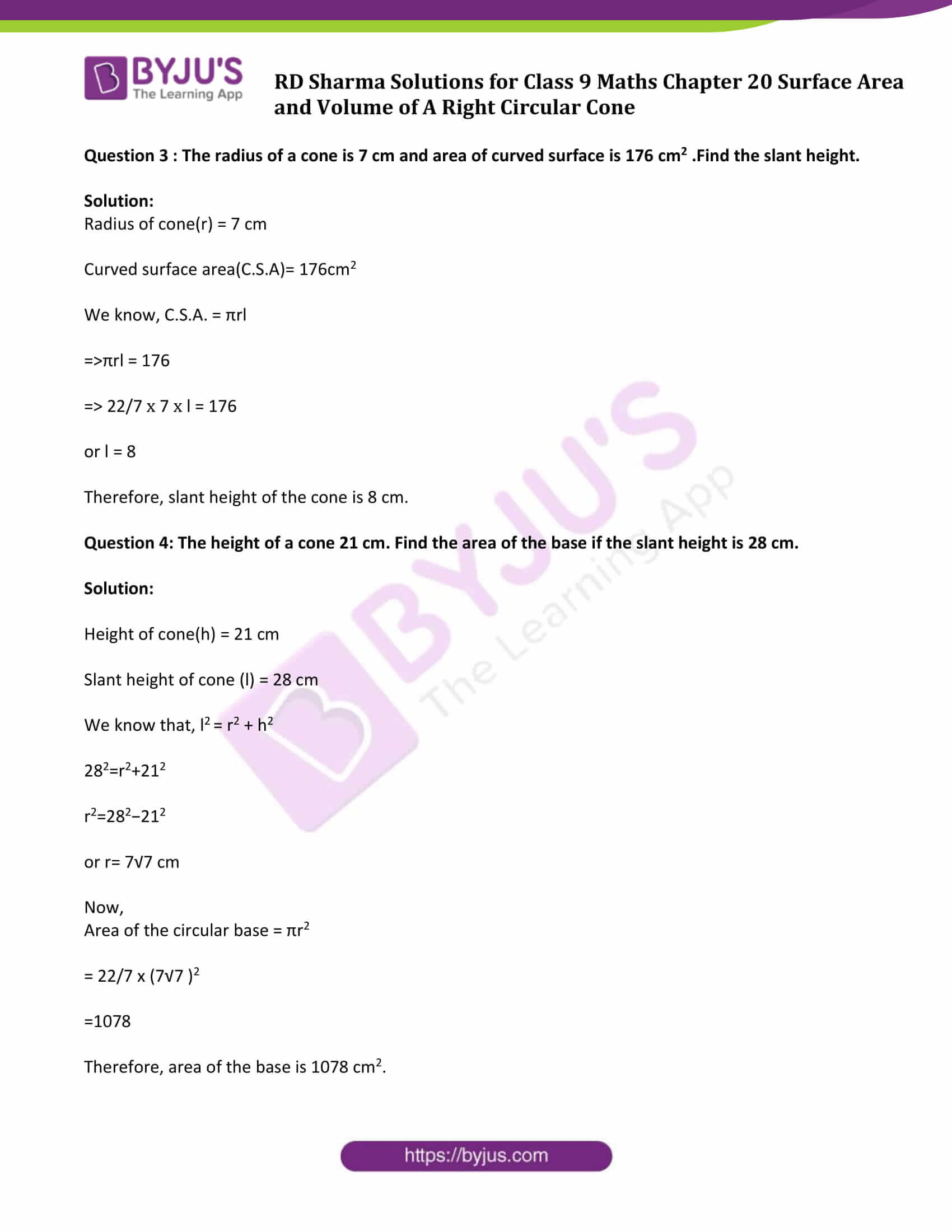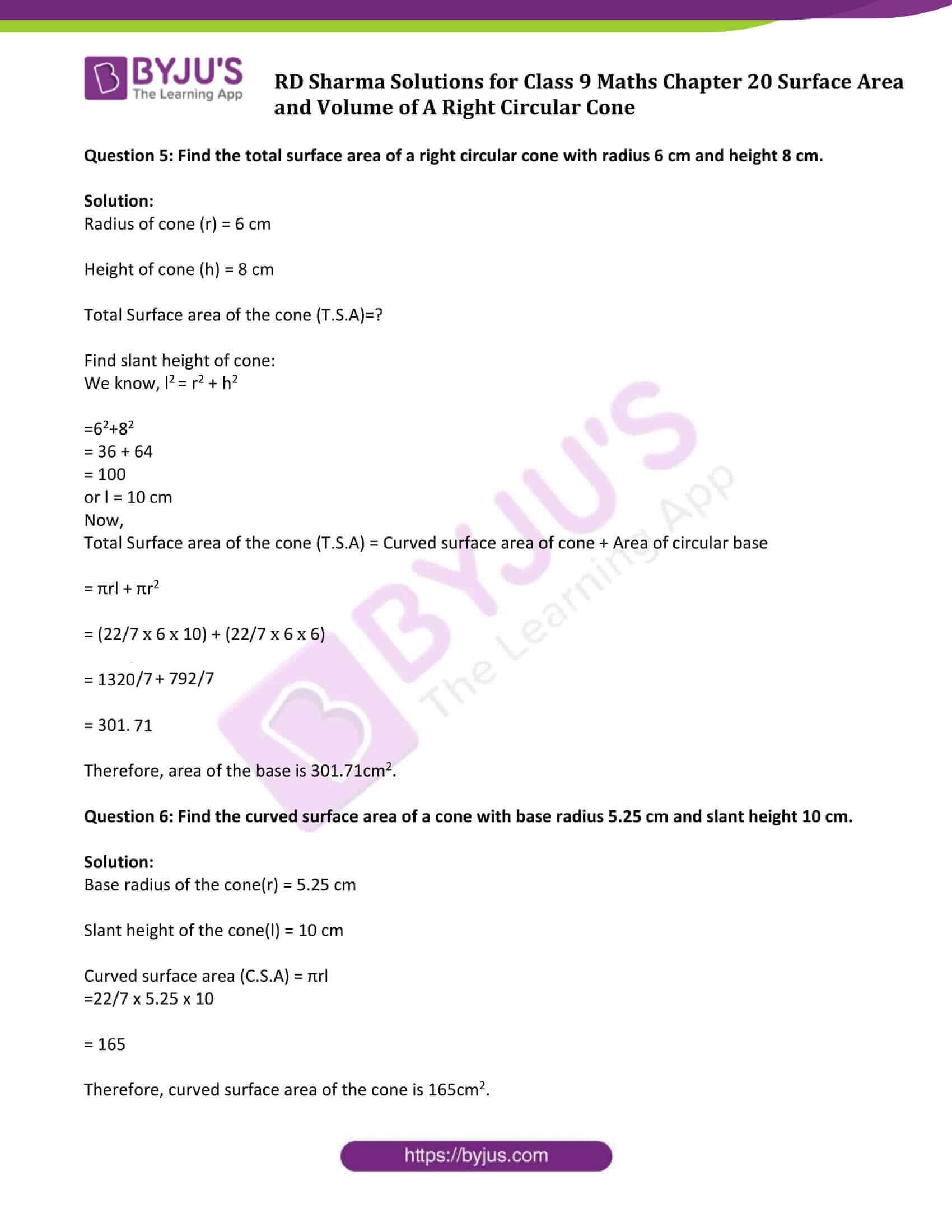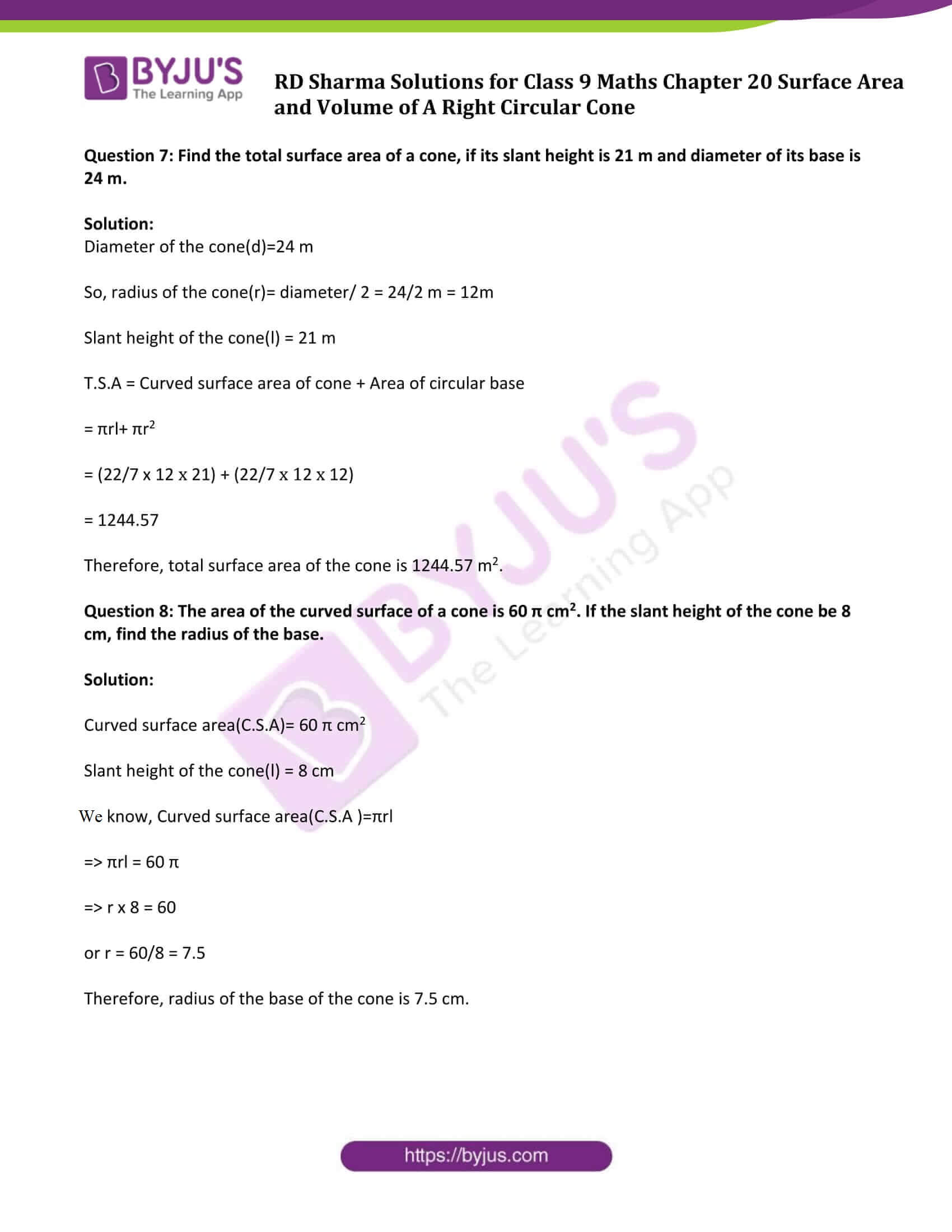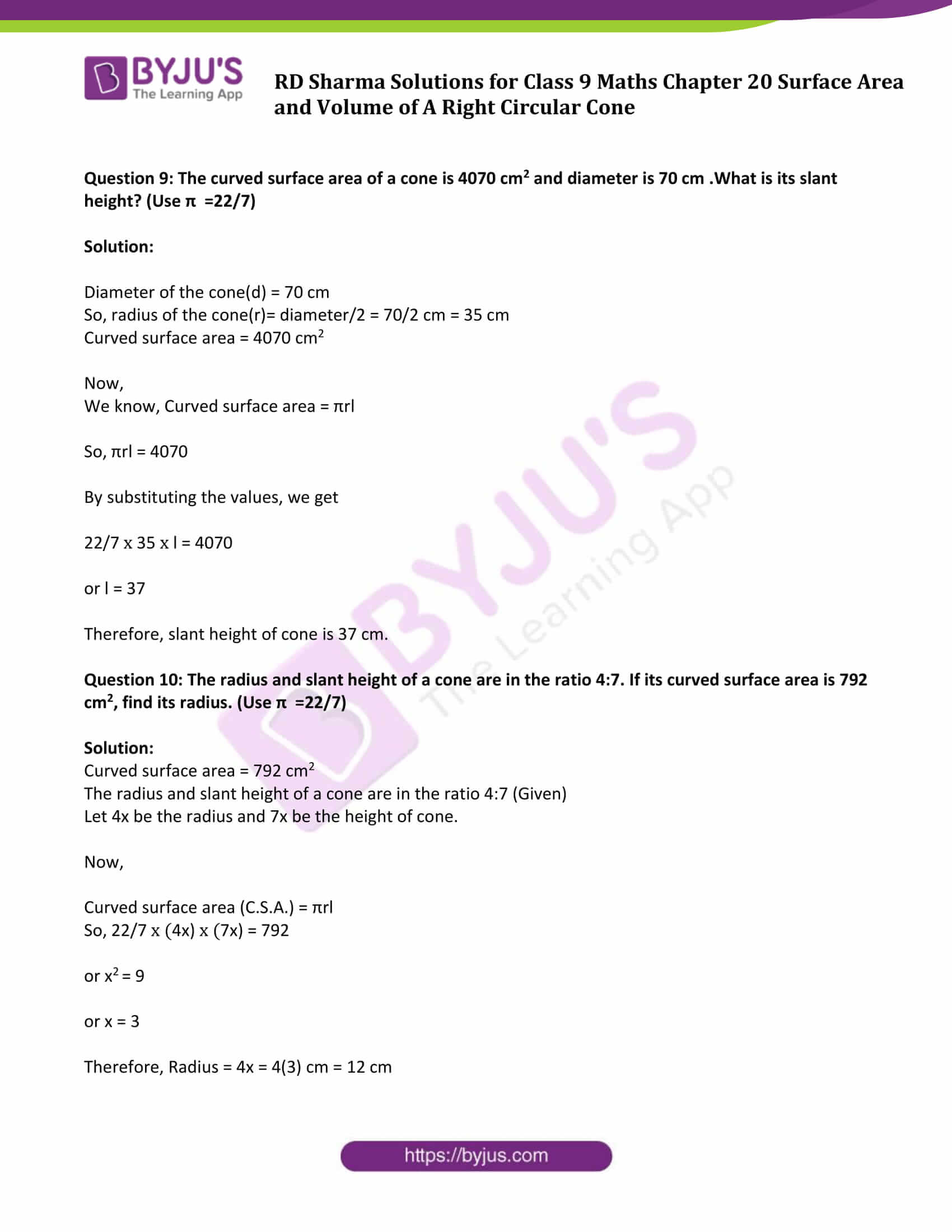### Access Answers to Maths RD Sharma Solutions for Class 9 Chapter 20 Surface Area and Volume of A Right Circular Cone Exercise 20.1 Page number 20.7

Question 1: Find the curved surface area of a cone, if its slant height is 60 cm and the radius of its base is 21 cm.

Solution:

Slant height of cone (l) = 60 cm

Radius of the base of the cone (r) = 21 cm

Now,

Curved surface area of the right circular cone = πrl = 22/7 x 21 x 60 = 3960 cm2

Therefore the curved surface area of the right circular cone is 3960 cm2

Question 2: The radius of a cone is 5cm and vertical height is 12cm. Find the area of the curved surface.

Solution:

Radius of cone (r) = 5 cm

Height of cone (h) = 12 cm

Find Slant Height of cone (l):

We know, l2 = r2 + h2

l2 = 52 +122

l2 = 25 + 144 = 169

Or l = 13 cm

Now,

C.S.A = πrl =3.14 x 5 x 13 = 204.28

Therefore, the curved surface area of the cone is 204.28 cm2

Question 3 : The radius of a cone is 7 cm and area of curved surface is 176 cm2 .Find the slant height.

Solution:

Radius of cone(r) = 7 cm

Curved surface area(C.S.A)= 176cm2

We know, C.S.A. = πrl

⇒πrl = 176

⇒ 22/7 x 7 x l = 176

or l = 8

Therefore, slant height of the cone is 8 cm.

Question 4: The height of a cone 21 cm. Find the area of the base if the slant height is 28 cm.

Solution:

Height of cone(h) = 21 cm

Slant height of cone (l) = 28 cm

We know that, l2 = r2 + h2

282=r2+212

r2=282−212

or r= 7√7 cm

Now,

Area of the circular base = πr2

= 22/7 x (7√7 )2

=1078

Therefore, area of the base is 1078 cm2.

Question 5: Find the total surface area of a right circular cone with radius 6 cm and height 8 cm.

Solution:

Radius of cone (r) = 6 cm

Height of cone (h) = 8 cm

Total Surface area of the cone (T.S.A)=?

Find slant height of cone:

We know, l2 = r2 + h2

=62+82

= 36 + 64

= 100

or l = 10 cm

Now,

Total Surface area of the cone (T.S.A) = Curved surface area of cone + Area of circular base

= πrl + πr2

= (22/7 x 6 x 10) + (22/7 x 6 x 6)

= 1320/7 + 792/7

= 301.71

Therefore, area of the base is 301.71cm2.

Question 6: Find the curved surface area of a cone with base radius 5.25 cm and slant height 10 cm.

Solution:

Base radius of the cone(r) = 5.25 cm

Slant height of the cone(l) = 10 cm

Curved surface area (C.S.A) = πrl

=22/7 x 5.25 x 10

= 165

Therefore, curved surface area of the cone is 165cm2.

Question 7: Find the total surface area of a cone, if its slant height is 21 m and diameter of its base is 24 m.

Solution:

Diameter of the cone(d)=24 m

So, radius of the cone(r)= diameter/ 2 = 24/2 m = 12m

Slant height of the cone(l) = 21 m

T.S.A = Curved surface area of cone + Area of circular base

= πrl+ πr2

= (22/7 x 12 x 21) + (22/7 x 12 x 12)

= 1244.57

Therefore, total surface area of the cone is 1244.57 m2.

Question 8: The area of the curved surface of a cone is 60 π cm2. If the slant height of the cone be 8 cm, find the radius of the base.

Solution:

Curved surface area(C.S.A)= 60 π cm2

Slant height of the cone(l) = 8 cm

We know, Curved surface area(C.S.A )=πrl

⇒ πrl = 60 π

⇒ r x 8 = 60

or r = 60/8 = 7.5

Therefore, radius of the base of the cone is 7.5 cm.

Question 9: The curved surface area of a cone is 4070 cm2 and diameter is 70 cm .What is its slant height? (Use π =22/7)

Solution:

Diameter of the cone(d) = 70 cm

So, radius of the cone(r)= diameter/2 = 70/2 cm = 35 cm

Curved surface area = 4070 cm2

Now,

We know, Curved surface area = πrl

So, πrl = 4070

By substituting the values, we get

22/7 x 35 x l = 4070

or l = 37

Therefore, slant height of cone is 37 cm.

Question 10: The radius and slant height of a cone are in the ratio 4:7. If its curved surface area is 792 cm2, find its radius. (Use π =22/7)

Solution:

Curved surface area = 792 cm2

The radius and slant height of a cone are in the ratio 4:7 (Given)

Let 4x be the radius and 7x be the height of cone.

Now,

Curved surface area (C.S.A.) = πrl

So, 22/7 x (4x) x (7x) = 792

or x2 = 9

or x = 3

Therefore, Radius = 4x = 4(3) cm = 12 cm

## RD Sharma Solutions for Class 9 Maths Chapter 20 Surface Area and Volume of A Right Circular Cone Exercise 20.1

RD Sharma Solutions Class 9 Maths Chapter 20 Surface Area and Volume of A Right Circular Cone Exercise 20.1 is based on the following topics:

• Right Circular Cone Introduction
• Surface Area of a Right Circular Cone

## Frequently Asked Questions on RD Sharma Solutions for Class 9 Maths Chapter 20

### Why should we follow RD Sharma Solutions for Class 9 Maths Chapter 20?

RD Sharma Solutions for Class 9 Maths Chapter 20 is the best reference material that offers complete and quality information about different Math concepts. The questions that have been given in the solutions have been solved in an easy-to-remember format, which further helps students to clearly understand and remember the answers. So, it’s clear that RD Sharma Textbooks for Class 9 are essential reference books to score high in examinations. To score good marks, practising these solutions for Class 9 Maths can help to a great extent.

### How BYJU’S RD Sharma Solutions for Class 9 Maths Chapter 20 help the students in preparing for board exams?

Our solution module uses various examples and diagrams to explain the questions, wherever necessary. For CBSE board students aiming at securing an excellent score, solving RD Sharma Solutions for Class 9 is a must. RD Sharma Solutions for Class 9 Maths Chapter 20 helps the students in gaining a better knowledge of the topics covered. Solving the questions from each exercise will ensure that the students score high marks in the exams.

### What are the topics covered under RD Sharma Solutions for Class 9 Maths Chapter 20?

RD Sharma Solutions for Class 9 Maths Chapter 20 includes the following topics:
1. Right Circular Cone Introduction
2. Surface Area of a Right Circular Cone